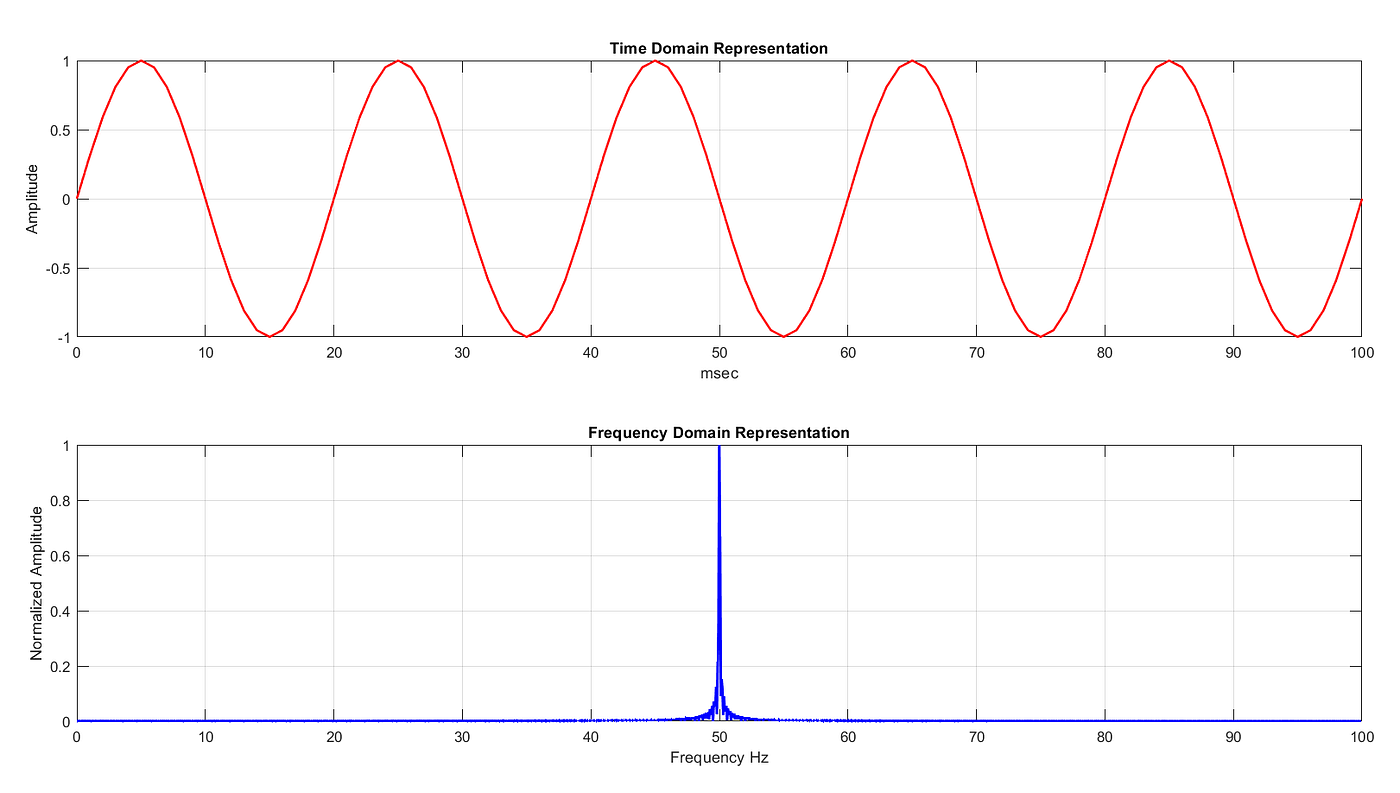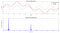# Demystifying the Fourier transform

Fourier transform is the essence of modern data analysis and one of the beauties of mathematics. Fourier transform is a mathematical bridge that joins the time and frequency domain of signal or function representation. If you apply Fourier transform on a function in time domain, you get another function that exists in frequency domain, equivalent to the original time-domain function and vice-versa.

What do time and frequency domains of representation mean?

As shown in the figure below, a sine wave is represented in the both time and frequency domain.Figure 1. Time and frequency domain representation of a signal with a single frequency

In time-domain representation, the signal has variation in amplitude over time i.e. amplitude is a function of time, whereas in the frequency domain the signal has variation in amplitude over frequency i.e. amplitude is a function of frequency. As there is only one sine wave, in the frequency domain the signal has a peak at 50Hz, and the rest are approximately zero. Also, this can be cross verified with a time scale from the time-domain graph. 1 cycle per 20 msec is equivalent to a 50Hz frequency signal.

Another example of signal representation is given below.Figure 2. Time and frequency domain representation of a signal with multiple frequencies

In the figure above, There are two different sine waves (dotted lines) added together to create a third wave (solid red line). In frequency domain representation there are two peaks for two different sine waves. The peak at 10 and 50 Hz has a normalized amplitude of unity and 0.5 respectively. This shows the amplitude vs frequency relation of a time-domain signal. The time scale can be verified similarly to 1st example to cross verify the representations.

Fourier Series: Let’s compose the signal.

The fundamental idea behind the Fourier transform lies in the Fourier Series. Fourier theorem states that any periodic function can be represented as a weighted sum of sine and cosine functions. Whereas the weight coefficients (of the Fourier series) decide the contribution of each sine and cosine component to construct the original periodic function.

What do weight coefficients mean?
A sine wave is a periodic signal. Any sine wave can be mathematically described as given in Eq1. Where, Em is the peak amplitude, ωt is an angular frequency of a rotating vector, ϕ is the phase angle and B is the DC component on which sinusoidal oscillations are superimposed. In the Fourier series, each sinusoidal component is set by the coefficients such that their summation in the time domain produces the desired signal.

Let’s visualize the above-mentioned Fourier theorem. As shown in Figure 3. When 5 different sine waves are added in the time domain, the summation produces another periodic signal. The Fourier series tells the properties of a resultant periodic function. So in essence, the Fourier series composes the signal.

Fourier Transform: Let’s decompose the signal.

From the Fourier theorem, it is quite clear that any periodic function can be constructed from a weighted summation of multiple sine and cosine functions. But what if we have a periodic signal as shown in figure 3. (Black -Composed) and asked to find its frequency components and their weights. This is what Fourier transform does, it decomposes the signal and tells what frequencies are present in the signal with their respective magnitudes and phase angles.

Let's visualize the Fourier transform of the periodic signal as shown in figure 3 (Black -Composed). As we have constructed this signal from 5 different sine waves, we must get 5 different spikes in the frequency domain with their respective magnitude.

Ok, so we have got the expected result. Till now, we have only talked about the consequences of mathematical operations that we call Fourier transform. But what is the magical thing that happens inside a black box of Fourier transform that finds the signals from which the original signal is constructed?

Demystifying the Fourier Transform

Let’s first have a look at the equation of Fourier transform.

This equation may seem difficult when you see integral and exponent. But the thought behind this equation is quite simple yet astonishing. f(x) is a time-domain function, multiplied with some exponential term and integrated over -∞ to +∞. Before understanding the actual meaning of the exponential term. Let’s have some brainstorming to know why it is there.
Imagine, your friend asks you to choose any 1 number between 1 to 10 and then he runs a so-called scanning mechanism to know what you have chosen. He will ask you to compare the number with each number from the specified range and revert where it matches. So to speak this is what a Fourier transform does, it finds a correlation between f(x) and sine, cosine functions in the range of -∞ to +∞.
Similarly, if a periodic function f(x) is multiplied with other periodic functions and their sum of product is plotted as a function of frequency f(ξ) you will get to know about the periodic properties of the original function f(x) in terms of frequency. Again let’s have a graphical representation of this.

As shown in the figure above, the time domain signal is a pure sine wave of 5Hz. Different sinusoids from the bunch are multiplied and the sum of products is plotted for respective frequency in the 3rd subplot of figure 5. As a result, a peak at 5Hz is visible, and the rest are approximately zero. This is an intuitive but very basic, low-frequency resolution version of Fourier transform.

What is the significance of that exponential term?

The answer to this question lies in the complex number and Euler's formula.

Euler’s formula states the fundamental relation between trigonometric and complex exponential functions. Multiplying anything with e^ix is equivalent to have a rotation on a unit circle represented on a 2D complex plane. As a result, it creates a bunch of complex sinusoids which gets multiplied with the time domain signal and the sum of product is the Fourier transform for that respective frequency (ξ from Eq3). Derivation of Euler’s formula could be explored as a curiosity to know how exponential and trigonometric functions are related.
The output of the Fourier transform is a complex function as the time-domain function gets multiply with the complex exponential function. Amplitude and phase can be extracted as a function of frequency by taking the magnitude and angle of output complex function of Fourier transform.

The great physicist Richard Feynman called the Euler’s identity “the most remarkable formula in mathematics.”

Like a Shakespearean sonnet that captures the very essence of love, or a painting that brings out the beauty of the human form that is far more than just skin deep, Euler’s equation reaches down into the very depths of existence
- Prof. Keith Devlin

Discrete Fourier Transform and Fast Fourier Transform

So far we have seen the continuous functions of time. But when it comes to recording and processing signals from the physical world, things have to get into a discrete domain. DFT is equivalent to Fourier transform but in the discrete domain where finite data samples are recorded with a fixed time interval. FFT is a computationally efficient extension of DFT. It is more about an algorithmic approach towards DFT to have a less computational burden on data processing systems than something fundamentally constructed mathematical operation.

Limitations of Fourier Transform

1. By fundamental limit of uncertainty, it can not provide simultaneous time and frequency localization. To know a highly certain spectrum of a signal, a long-duration time-domain signal is required. Hence it is not suitable for non-stationary signals.
2. Fourier transform can not completely resolve information when periodic oscillations are superimposed on decaying signals.

Electronic Engineer, Aerospace Enthusiastic, Amateur Astronomer

## More from Aniket kamat

Electronic Engineer, Aerospace Enthusiastic, Amateur Astronomer